Mathematical Induction - Questions and Solutions

Mathematical Induction
Serial order wise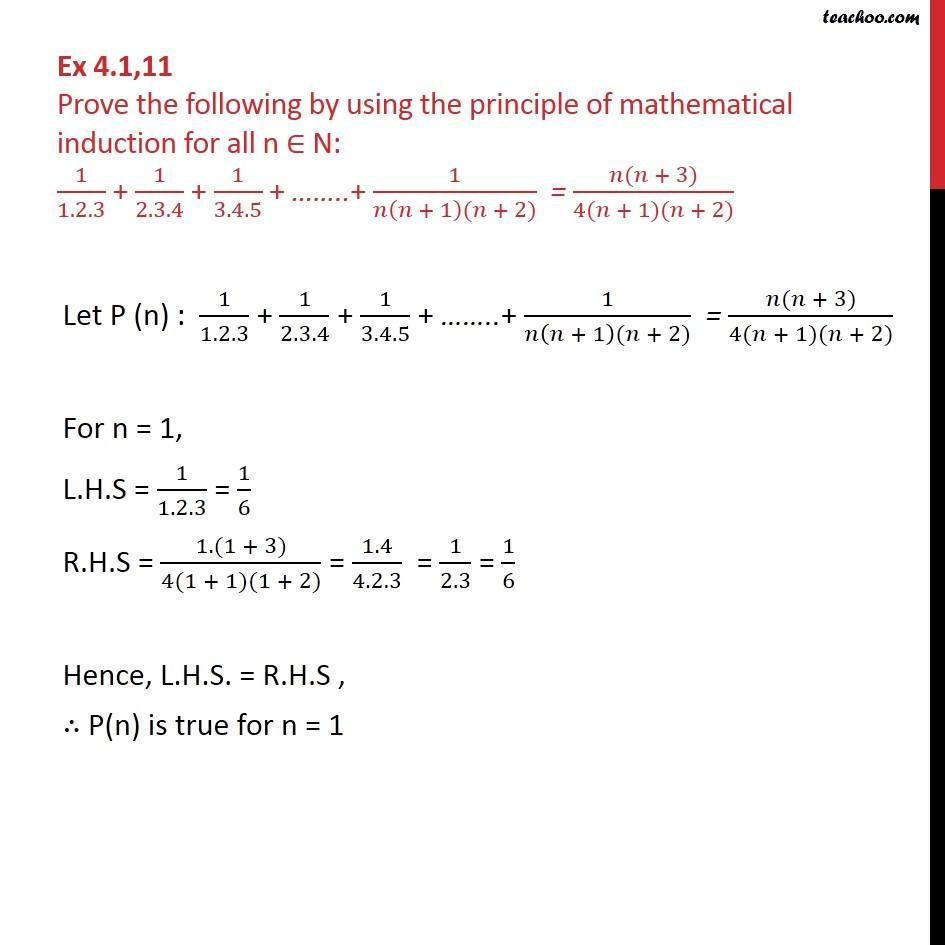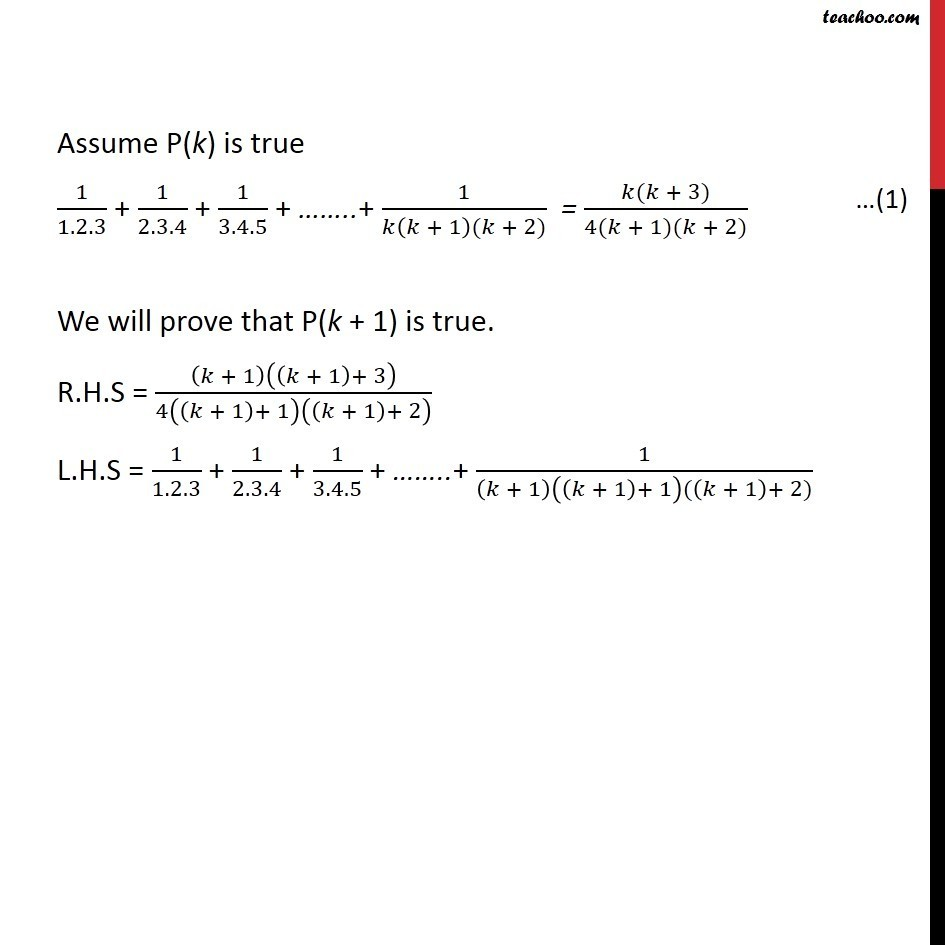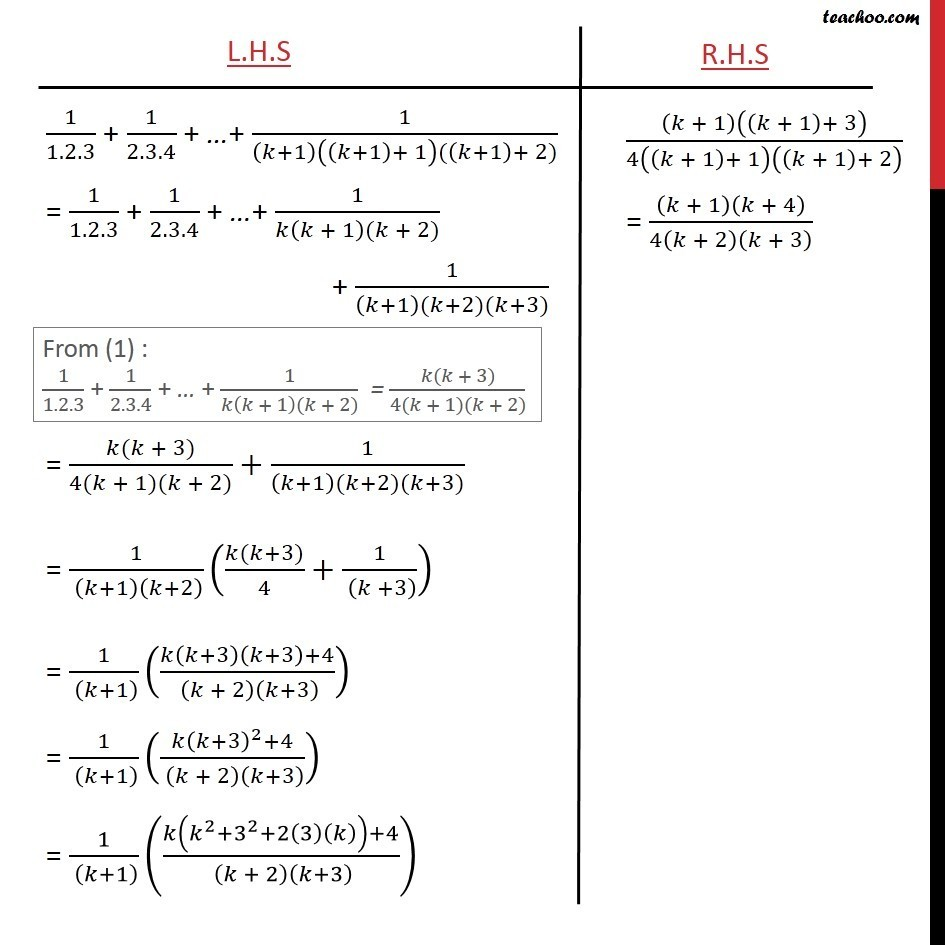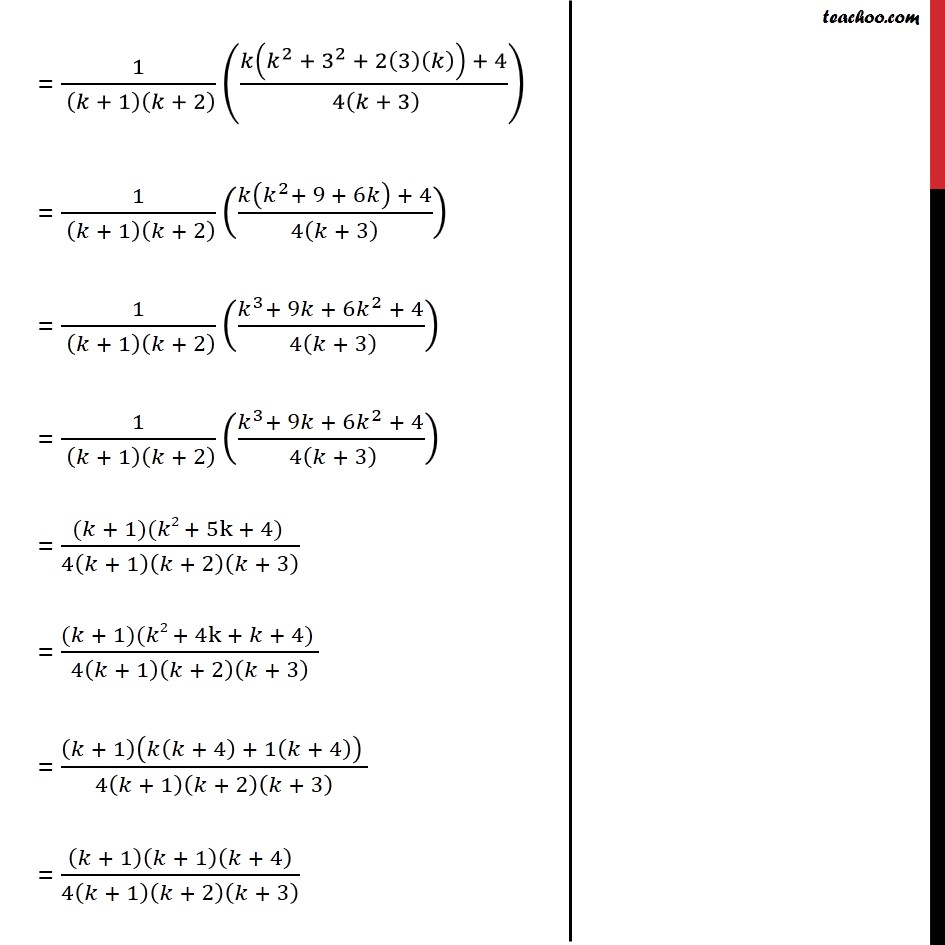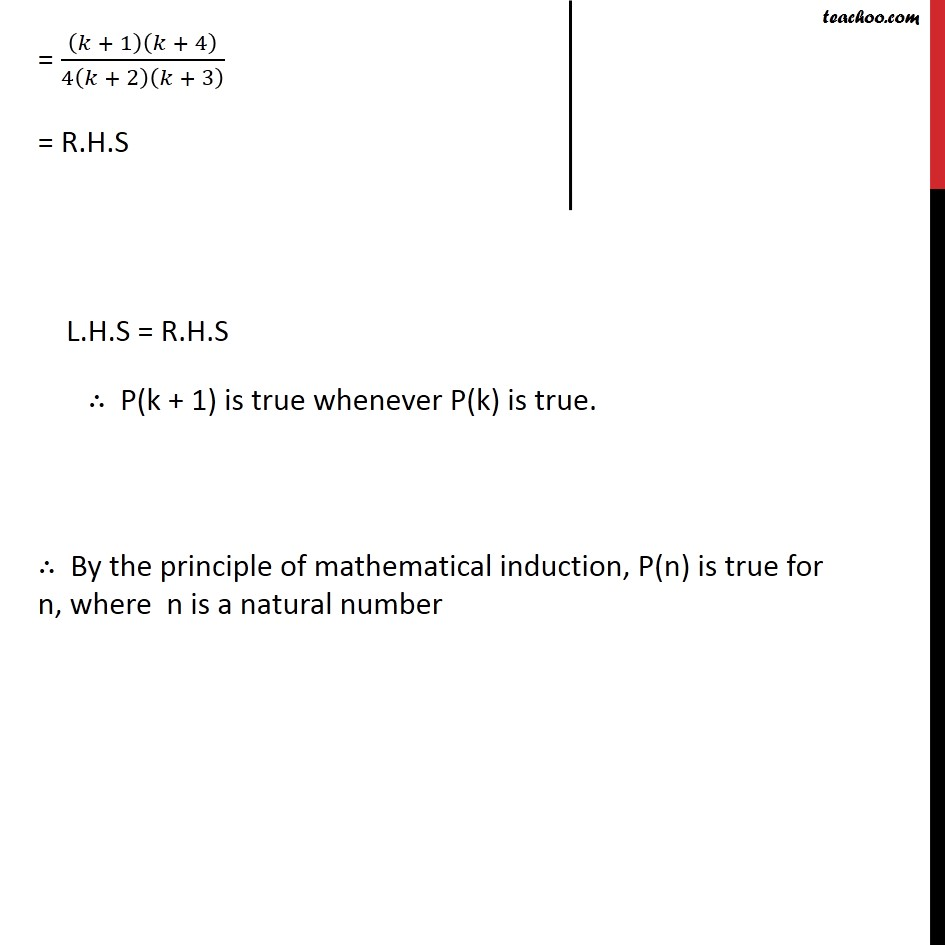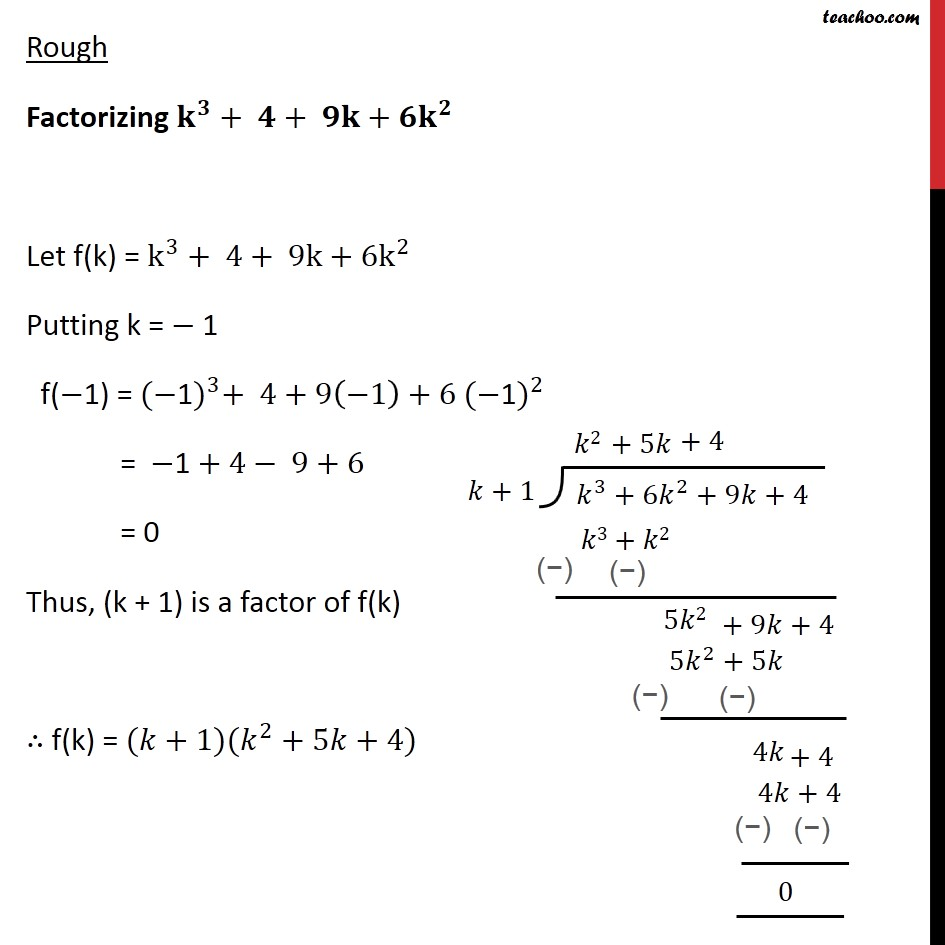Learn in your speed, with individual attention - Teachoo Maths 1-on-1 Class

### Transcript

Question11 Prove the following by using the principle of mathematical induction for all n ∈ N: 1﷮1.2.3﷯ + 1﷮2.3.4﷯ + 1﷮3.4.5﷯ + ……..+ 1﷮𝑛 𝑛 + 1﷯(𝑛 + 2)﷯ = 𝑛(𝑛 + 3)﷮4(𝑛 + 1)(𝑛 + 2)﷯ Let P (n) : 1﷮1.2.3﷯ + 1﷮2.3.4﷯ + 1﷮3.4.5﷯ + ……..+ 1﷮𝑛 𝑛 + 1﷯(𝑛 + 2)﷯ = 𝑛(𝑛 + 3)﷮4(𝑛 + 1)(𝑛 + 2)﷯ For n = 1, L.H.S = 1﷮1.2.3﷯ = 1﷮6﷯ R.H.S = 1.(1 + 3)﷮4(1 + 1)(1 + 2)﷯ = 1.4﷮4.2.3﷯ = 1﷮2.3﷯ = 1﷮6﷯ Hence, L.H.S. = R.H.S , ∴ P(n) is true for n = 1 Assume P(k) is true 1﷮1.2.3﷯ + 1﷮2.3.4﷯ + 1﷮3.4.5﷯ + ……..+ 1﷮𝑘 𝑘 + 1﷯(𝑘 + 2)﷯ = 𝑘(𝑘 + 3)﷮4(𝑘 + 1)(𝑘 + 2)﷯ We will prove that P(k + 1) is true. R.H.S = 𝑘 + 1﷯ 𝑘 + 1﷯+ 3﷯﷮4 𝑘 + 1﷯+ 1﷯ 𝑘 + 1﷯+ 2﷯﷯ L.H.S = 1﷮1.2.3﷯ + 1﷮2.3.4﷯ + 1﷮3.4.5﷯ + ……..+ 1﷮ 𝑘 + 1﷯ 𝑘 + 1﷯+ 1﷯( 𝑘 + 1﷯+ 2)﷯ Rough Factorizing 𝐤﷮𝟑﷯+ 𝟒+ 𝟗𝐤+ 𝟔𝐤﷮𝟐﷯ Let f(k) = k﷮3﷯+ 4+ 9k+ 6k﷮2﷯ Putting k = − 1 f(−1) = (−1)﷮3﷯+ 4+9 −1﷯+6 (−1)﷮2﷯ = −1 + 4− 9+6 = 0 Thus, (k + 1) is a factor of f(k) ∴ f(k) = (𝑘+1)( 𝑘﷮2﷯+5𝑘+4)